Fifth Chapter Lesson-7 Loop Control Statement Related algorithm & flowchart.

At the end of this lesson-

• 1. You will be able to write algorithm and flowchart for printing numbers from 1 to 10. Or
• You will be able to write algorithm and flowchart for printing the 1 2 3 4 5 6 7 8 9 10 series.
• 2. You will be able to write algorithm and flowchart for printing numbers from 1 to n. Or
• You will be able to write algorithm and flowchart for printing the 1 2 3 4 – – – – n series.
• 3. You will be able to write algorithm and flowchart for printing odd numbers from 1 to 10. Or
• You will be able to write algorithm and flowchart for printing the 1 3 5 7 9 series.
• 4. You will be able to write algorithm and flowchart for printing odd numbers from 1 to n. Or
• You will be able to write algorithm and flowchart for printing the 1 3 5 7- – – n series.
• 5. You will be able to write algorithm and flowchart for printing even numbers from 1 to 10. Or
• You will be able to write algorithm and flowchart for printing the 2 4 6 8 10 series.
• 6. You will be able to write algorithm and flowchart for printing even numbers from 1 to n. Or
• You will be able to write algorithm and flowchart for printing the 2 4 6 – – – n series.
• 7. You will be able to write algorithm and flowchart to calculate the sum of numbers from 1 to 100. Or
• You will be able to write algorithm and flowchart to calculate the sum of the 1+2+3+ – – +100 series.
• 8. You will be able to write algorithm and flowchart to calculate the sum of numbers from 1 to n. Or
• You will be able to write algorithm and flowchart to calculate the sum of the 1+2+3+ – – +n series.
• 9. You will be able to write algorithm and flowchart to calculate the sum of odd numbers from 1 to 100. Or
• You will be able to write algorithm and flowchart to calculate the sum of the 1+3+5+ – – +100 series.
• 10. You will be able to write algorithm and flowchart to calculate the sum of even numbers from 1 to 100. Or
• You will be able to write algorithm and flowchart to calculate the sum of the 2+4+6+ – – +100 series.

1. Algorithm and flowchart for printing numbers from 1 to 10. Or

Algorithm and flowchart for printing the 1 2 3 4 5 6 7 8 9 10 series.

• Algorithm
• Step-1: Start
• Step-2: Let i=1
• Step-3: If (i ≤ 10), then go to step-4,
•           Otherwise go to step-6
• Step-4: Print value of i
• Step-5: i=i+1 and again go to step-3
• Step-6: Stop2. Algorithm and flowchart for printing numbers from 1 to n. Or

Algorithm and flowchart for printing the 1 2 3 4 – – – – n series.

• Algorithm
• Step-1: Start
• Step-2: Input a number in variable n
• Step-3: Let i=1
• Step-4: If (i ≤ n), then go to step-5,
•          Otherwise go to step-7
• Step-5: Print value of i
• Step-6: i=i+1 and again go to step-4
• Step-7: Stop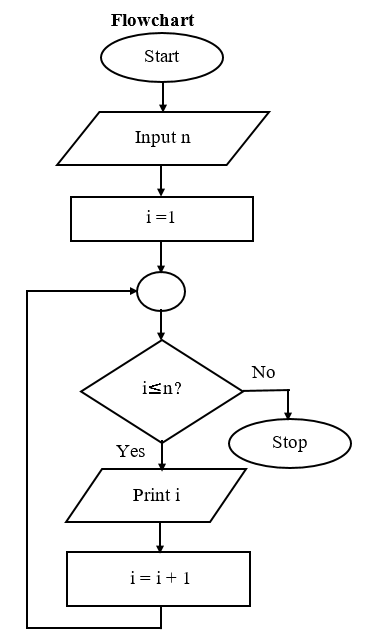3. Algorithm and flowchart for printing odd numbers from 1 to 10. Or

Algorithm and flowchart for printing the 1 3 5 7 9 series.

• Algorithm
• Step-1: Start
• Step-2: Let i=1
• Step-3: If (i ≤ 10), then go to step-4,
•          Otherwise go to step-6
• Step-4: Print value of i
• Step-5: i=i+2 and again go to step-3
• Step-6: Stop4. Algorithm and flowchart for printing odd numbers from 1 to n. Or

Algorithm and flowchart for printing the 1 3 5 7- – – n series.

• Algorithm
• Step-1: Start
• Step-2: Input a number in variable n
• Step-3: Let i=1
• Step-4: If (i ≤ n), then go to step-5,
•          Otherwise go to step-7
• Step-5: Print value of i
• Step-6: i=i+2 and again go to step-4
• Step-7:Stop5. Algorithm and flowchart for printing even numbers from 1 to 10. Or

Algorithm and flowchart for printing the 2 4 6 8 10 series.

• Algorithm
• Step-1: Start
• Step-2: Let i=2
• Step-3: If (i ≤ 10), then go to step-4,
•            Otherwise go to step-6
• Step-4: Print value of i
• Step-5: i=i+2 and again go to step-3
• Step-6: Stop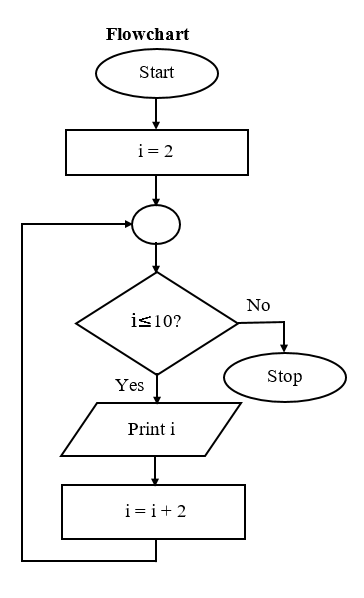6. Algorithm and flowchart for printing even numbers from 1 to n. Or

Algorithm and flowchart for printing the 2 4 6 – – – n series.

• Algorithm
• Step-1: Start
• Step-2: Input a number in variable n
• Step-3: Let i=2
• Step-4: If (i ≤ n), then go to step-5
•           Otherwise go to step-7
• Step-5: Print value of i
• Step-6: i=i+2 and again go to step-4
• Step-7: Stop7. Algorithm and flowchart to calculate the sum of numbers from 1 to 100. Or

Algorithm and flowchart to calculate the sum of the 1+2+3+ – – +100 series.

• Algorithm
• Step-1: Start
• Step-2: Let i=1 and sum=0
• Step-3: If (i ≤ 100), then go to step-4,
•           Otherwise go to step-6
• Step-4: sum = sum + i
• Step-5: i=i+1 and again go to step-3
• Step-6: Print value of sum
• Step-7: Stop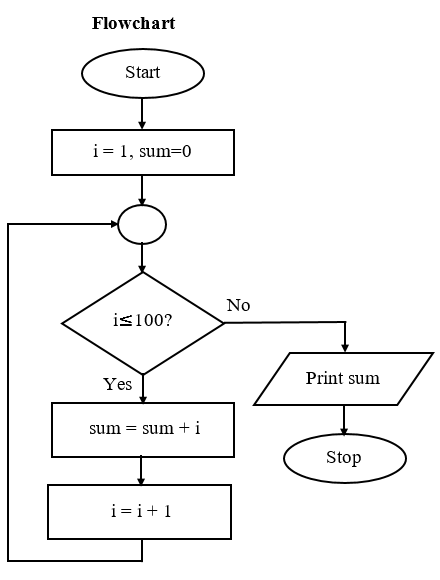8. Algorithm and flowchart to calculate the sum of numbers from 1 to n. Or

Algorithm and flowchart to calculate the sum of the 1+2+3+ – – +n series.

• Algorithm
• Step-1: Start
• Step-2: Input a number in variable n
• Step-3: Let i=1 and sum=0
• Step-4: If (i ≤ n), then go to step-5,
•           Otherwise go to step-7
• Step-5: sum = sum + i
• Step-6: i=i+1 and again go to step-4
• Step-7: Print value of sum
• Step-8: Stop9. Algorithm and flowchart to calculate the sum of odd numbers from 1 to 100. Or

Algorithm and flowchart to calculate the sum of the 1+3+5+ – – +100 series.

• Algorithm
• Step-1: Start
• Step-2: Let i=1 and sum=0
• Step-3: If (i ≤ 100), then go to step-4
•            Otherwise go to step-6
• Step-4: sum = sum + i
• Step-5: i=i+2 and go to again step-3
• Step-6: Print value of sum
• Step-7: Stop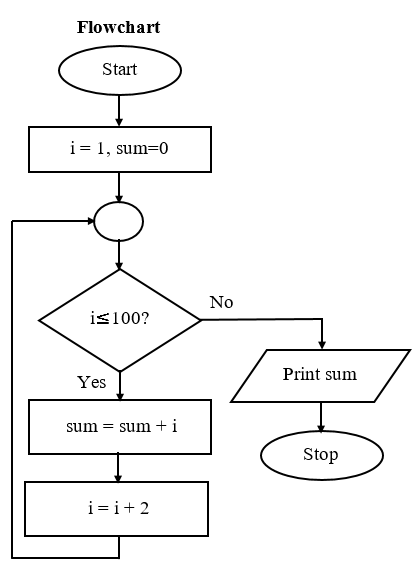10. Algorithm and flowchart to calculate the sum of even numbers from 1 to 100. Or

Algorithm and flowchart to calculate the sum of the 2+4+6+ – – +100 series.

• Algorithm
• Step-1: Start
• Step-2: Let i=2 and sum=0
• Step-3: If (i ≤ 100), then go to step-4,
•           Otherwise go to step-6
• Step-4: sum = sum + i
• Step-5: i=i+2 and again go to step-3
• Step-6: Print value of sum
• Step-7: Stop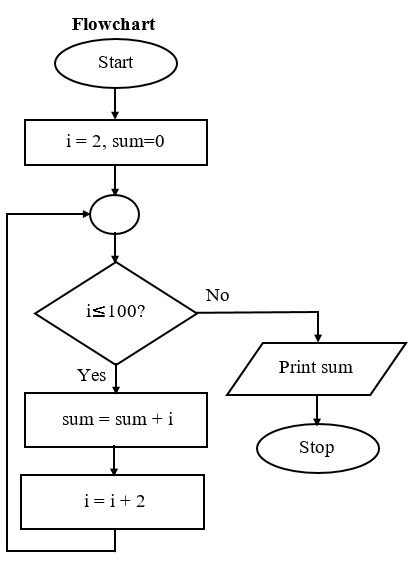Practice

• 1. Algorithm and flowchart for printing the 5 9 13 17 – – -n series.
• 2. Algorithm and flowchart for printing the 1 4 9 16 25 – – -n series.
• 3. Algorithm and flowchart for printing the 1 8 27 64 – – -n series.
• 4. Algorithm and flowchart for printing the 1 4 27 256 – – -n series.
• 5. Algorithm and flowchart to calculate the sum of odd numbers from 1 to n. Or
• Algorithm and flowchart to calculate the sum of the 1+3+5+ – – +n series.
• 6. Algorithm and flowchart to calculate the sum of even numbers from 1 to n. Or
• Algorithm and flowchart to calculate the sum of the 2+4+6+ – – +n series.
• 7. Algorithm and flowchart to calculate the sum of the 12+22+32+42+- – -+ n2 series.
• 8. Algorithm and flowchart to calculate the sum of the 13+23+33+43+- – -+ n3 series.
• 9. Algorithm and flowchart to calculate the sum of the 11+22+33+44+ – – -+nn series.
• 10. Algorithm and flowchart to calculate factorial of a positive number.

Lesson Evaluation-

Knowledge Based Questions:

Comprehension Based Questions:

Creative Questions:

Multiple Choice Questions:

Written by,